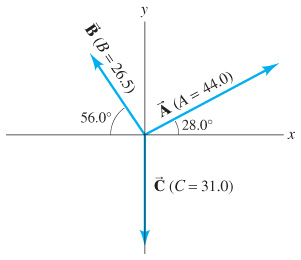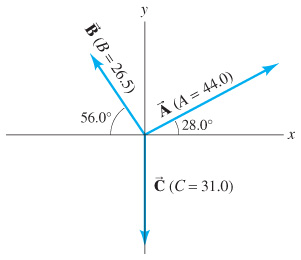Physics Practice Problems Vector Addition & Subtraction Practice Problems Solution: For the vectors shown in the figure, determine B -...

🤓 Based on our data, we think this question is relevant for Professor Munsell & Lewicki's class at PURDUE.

# Solution: For the vectors shown in the figure, determine B - 2A. Vector magnitudes are given in arbitrary units.

###### Problem

For the vectors shown in the figure, determine B - 2A. Vector magnitudes are given in arbitrary units.###### Solution

Whenever we're adding and subtracting vectors in 2D, we follow these steps:

1. Draw a diagram and resolve the vectors into components.
3. Add or subtract vectors as required.
4. Convert back to magnitude-angle notation (unless the problem only asks for components).

For step 1, we'll use these equations to find the x- and y-components of our given vectors:

For step 4, we'll use these equations to calculate the magnitude and angle from the +x-axis:

$\overline{)|\stackrel{⇀}{A}|{=}\sqrt{{{A}_{x}}^{2}+{{A}_{y}}^{2}}}$

Remember that if we solve the last equation for θ, we always have to check whether the result we get makes sense based on the components. We might need to add or subtract 180°, because the inverse tangent function only returns angles between −90° and 90°.

Step 1. For this problem, we're given a diagram, so we don't need to draw one from scratch. Unfortunately one of the given angles is measured clockwise from the negative x-axis, and we need  θB as a counterclockwise angle from the positive x-axis for our formulas to work:

${\theta }_{B}=180°-56.0°=\mathbf{124}\mathbf{°}$

Now we can plug in known information for $\stackrel{⇀}{B}$ and get its components, keeping an extra significant figure to reduce rounding error:

Since we're subtracting $2\stackrel{⇀}{A}$, let's just go ahead and find 2Ax and 2Ay:View Complete Written Solution#### Q. For the vectors given in the figure, determine(a) A - B + C(b) A + B - C(c) C - A - B

Solved • Mon Oct 29 2018 19:00:20 GMT-0400 (EDT)

#### Q. The summit of a mountain, 2450 m above base camp, is measured on a map to be 4580 m horizontally from the camp in a direction 32.4° west of north. Cho...

Solved • Mon Oct 29 2018 19:00:20 GMT-0400 (EDT)